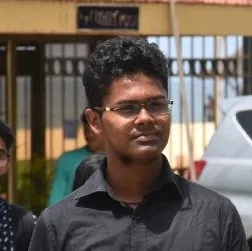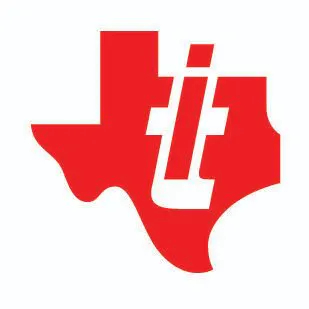Published

# Anti - Theft System with Gesture Unlock

A simple solution that solves the problem of leaving rooms unattended and hence, prevents robberies.

IntermediateProtip1,499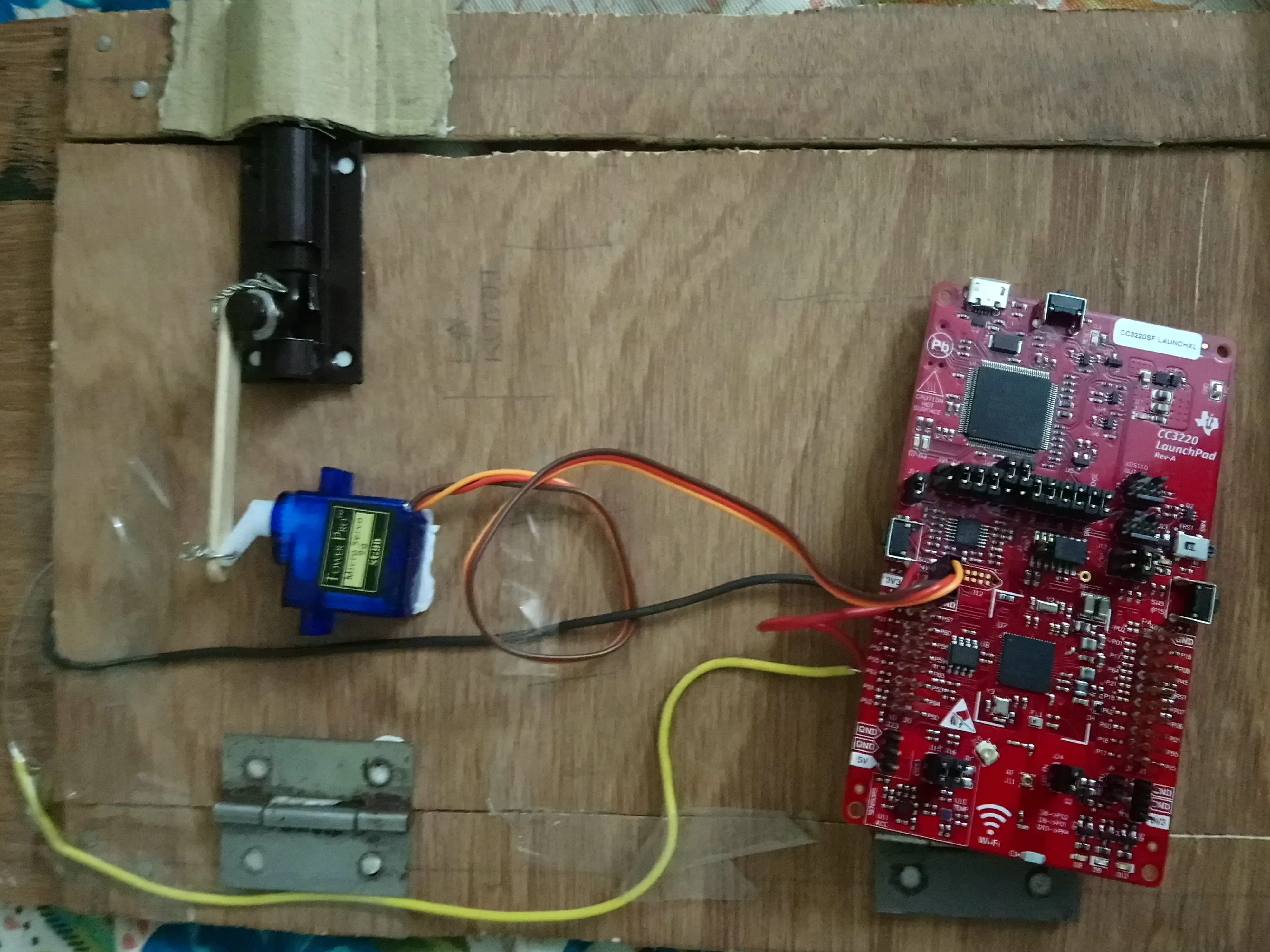## Things used in this project

### Hardware components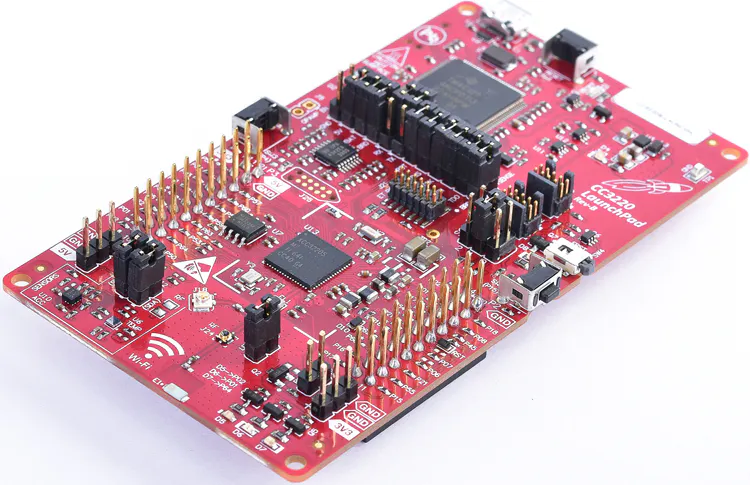×1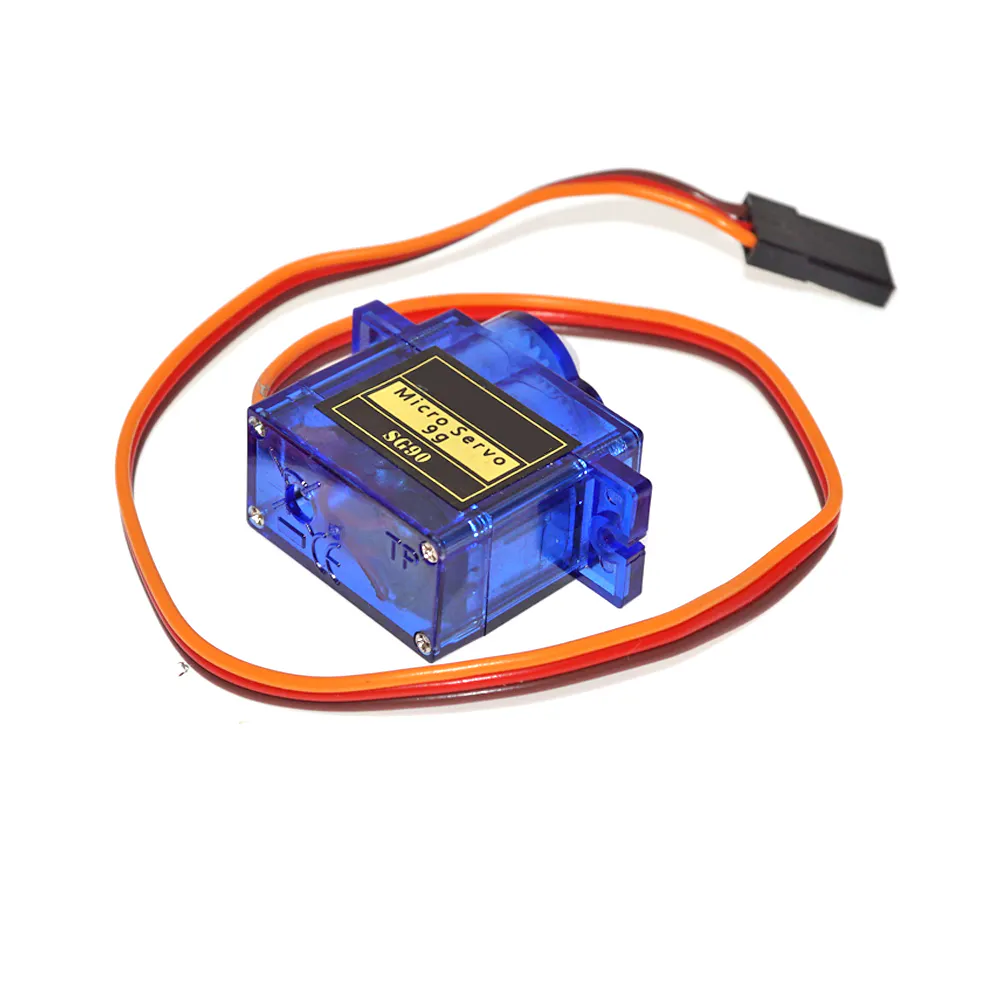SG90 Micro-servo motor
×1

### Software apps and online servicesTexas Instruments Energia

## Code

### Remote Unlock

C/C++
When the code runs, an IP address is given as output through which the user's phone can connect to the micro-controller.
```#include <WiFi.h>
int servo = 9;

int angle;
int pwm;

// your network name also called SSID
char ssid[] = "NITK-NET";
// your network key Index number (needed only for WEP)
int keyIndex = 0;

WiFiServer server(80);

void setup() {
Serial.begin(115200);      // initialize serial communication

pinMode(RED_LED, OUTPUT);      // set the LED pin mode
pinMode(servo, OUTPUT);
// attempt to connect to Wifi network:
Serial.print("Attempting to connect to Network named: ");
// print the network name (SSID);
Serial.println(ssid);
// Connect to WPA/WPA2 network. Change this line if using open or WEP network:
while ( WiFi.status() != WL_CONNECTED) {
// print dots while we wait to connect
Serial.print(".");
delay(300);
}

Serial.println("\nYou're connected to the network");

// print dots while we wait for an ip addresss
Serial.print(".");
delay(300);
}

// you're connected now, so print out the status
printWifiStatus();

Serial.println("Starting webserver on port 80");
server.begin();                           // start the web server on port 80
Serial.println("Webserver started!");
}

void loop() {
int i = 0;
WiFiClient client = server.available();   // listen for incoming clients

if (client) {                             // if you get a client,
Serial.println("new client");           // print a message out the serial port
char buffer = {0};                 // make a buffer to hold incoming data
while (client.connected()) {            // loop while the client's connected
if (client.available()) {             // if there's bytes to read from the client,
Serial.write(c);                    // print it out the serial monitor
if (c == '\n') {                    // if the byte is a newline character

// if the current line is blank, you got two newline characters in a row.
// that's the end of the client HTTP request, so send a response:
if (strlen(buffer) == 0) {
// and a content-type so the client knows what's coming, then a blank line:
client.println("HTTP/1.1 200 OK");
client.println("Content-type:text/html");
client.println();

// the content of the HTTP response follows the header:
client.println("<h1 align=center><font color=\"red\">Welcome to the CC3200 WiFi Web Server</font></h1>");
client.print("RED LED <button onclick=\"location.href='/H'\">HIGH</button>");
client.println(" <button onclick=\"location.href='/L'\">LOW</button><br>");

// The HTTP response ends with another blank line:
client.println();
// break out of the while loop:
break;
}
else {      // if you got a newline, then clear the buffer:
memset(buffer, 0, 150);
i = 0;
}
}
else if (c != '\r') {    // if you got anything else but a carriage return character,
buffer[i++] = c;      // add it to the end of the currentLine
}

// Check to see if the client request was "GET /H" or "GET /L":
if (endsWith(buffer, "GET /H")) {
digitalWrite(RED_LED, HIGH); // GET /H turns the LED on

for (angle = 0; angle <= 140; angle += 5)  {
servoPulse(servo, angle);  }
//for (angle = 140; angle >= 0; angle -= 5)  {
//        servoPulse(servo, angle);  }

}
if (endsWith(buffer, "GET /L")) {
digitalWrite(RED_LED, LOW); // GET /L turns the LED off
for (angle = 140; angle >= 0; angle -= 5)  {
servoPulse(servo, angle);  }

//myservo.write(0);
//delay(100);
}
}
}
// close the connection:
client.stop();
Serial.println("client disonnected");
}
}

//
//a way to check if one array ends with another array
//
boolean endsWith(char* inString, char* compString) {
int compLength = strlen(compString);
int strLength = strlen(inString);

//compare the last "compLength" values of the inString
int i;
for (i = 0; i < compLength; i++) {
char a = inString[(strLength - 1) - i];
char b = compString[(compLength - 1) - i];
if (a != b) {
return false;
}
}
return true;
}

void printWifiStatus() {
// print the SSID of the network you're attached to:
Serial.print("SSID: ");
Serial.println(WiFi.SSID());

Serial.println(ip);

// print the received signal strength:
Serial.println(" dBm");
// print where to go in a browser:
Serial.println(ip);
}

void servoPulse (int servo, int angle)
{
pwm = (angle*11) + 500;      // Convert angle to microseconds
digitalWrite(servo, HIGH);
delayMicroseconds(pwm);
digitalWrite(servo, LOW);
delay(50);                   // Refresh cycle of servo
}
```

### Gesture Detection

Python
When the code runs, it outputs the expected hand sign, which when shown is used to unlock the room.
```	# Imports
import numpy as np
import cv2
import math
import serial
import time
import random

# Open Camera
capture = cv2.VideoCapture(0)
CCSerial = serial.Serial('com7', 115200)
time.sleep(2)

label1 = random.randint(1,5)

print(label1)
arr = []
while len(arr) < 200:

# Capture frames from the camera

# Get hand data from the rectangle sub window
cv2.rectangle(frame, (100, 100), (300, 300), (0, 255, 0), 0)
crop_image = frame[100:300, 100:300]

# Apply Gaussian blur
blur = cv2.GaussianBlur(crop_image, (3, 3), 0)

# Change color-space from BGR -> HSV
hsv = cv2.cvtColor(blur, cv2.COLOR_BGR2HSV)

# Create a binary image with where white will be skin colors and rest is black
mask2 = cv2.inRange(hsv, np.array([2, 0, 0]), np.array([20, 255, 255]))

# Kernel for morphological transformation
kernel = np.ones((5, 5))

# Apply morphological transformations to filter out the background noise
erosion = cv2.erode(dilation, kernel, iterations=1)

# Apply Gaussian Blur and Threshold
filtered = cv2.GaussianBlur(erosion, (3, 3), 0)
ret, thresh = cv2.threshold(filtered, 90, 255, 0)

# Show threshold image
cv2.imshow("Thresholded", thresh)

# Find contours
image, contours, hierarchy = cv2.findContours(thresh, cv2.RETR_TREE, cv2.CHAIN_APPROX_SIMPLE)

try:
# Find contour with maximum area
contour = max(contours, key=lambda x: cv2.contourArea(x))

# Create bounding rectangle around the contour
x, y, w, h = cv2.boundingRect(contour)
cv2.rectangle(crop_image, (x, y), (x + w, y + h), (0, 0, 255), 0)

# Find convex hull
hull = cv2.convexHull(contour)

# Draw contour
drawing = np.zeros(crop_image.shape, np.uint8)
cv2.drawContours(drawing, [contour], -1, (0, 255, 0), 0)
cv2.drawContours(drawing, [hull], -1, (0, 0, 255), 0)

# Find convexity defects
hull = cv2.convexHull(contour, returnPoints=False)
defects = cv2.convexityDefects(contour, hull)

# Use cosine rule to find angle of the far point from the start and end point i.e. the convex points (the finger
# tips) for all defects
count_defects = 0

for i in range(defects.shape):
s, e, f, d = defects[i, 0]
start = tuple(contour[s])
end = tuple(contour[e])
far = tuple(contour[f])

a = math.sqrt((end - start) ** 2 + (end - start) ** 2)
b = math.sqrt((far - start) ** 2 + (far - start) ** 2)
c = math.sqrt((end - far) ** 2 + (end - far) ** 2)
angle = (math.acos((b ** 2 + c ** 2 - a ** 2) / (2 * b * c)) * 180) / 3.14

# if angle > 90 draw a circle at the far point
if angle <= 90:
count_defects += 1
cv2.circle(crop_image, far, 1, [0, 0, 255], -1)

cv2.line(crop_image, start, end, [0, 255, 0], 2)
arr.append(count_defects)
# Print number of fingers
if count_defects == 1:
cv2.putText(frame, "ONE", (50, 50), cv2.FONT_HERSHEY_SIMPLEX, 2,(0,0,255),2)
elif count_defects == 2:
cv2.putText(frame, "TWO", (50, 50), cv2.FONT_HERSHEY_SIMPLEX, 2,(0,0,255), 2)
elif count_defects == 3:
cv2.putText(frame, "THREE", (5, 50), cv2.FONT_HERSHEY_SIMPLEX, 2,(0,0,255), 2)
elif count_defects == 4:
cv2.putText(frame, "FOUR", (50, 50), cv2.FONT_HERSHEY_SIMPLEX, 2,(0,0,255), 2)
elif count_defects == 5:
cv2.putText(frame, "FIVE", (50, 50), cv2.FONT_HERSHEY_SIMPLEX, 2,(0,0,255), 2)
else:
pass
except:
pass

# Show required images
cv2.imshow("Gesture", frame)
all_image = np.hstack((drawing, crop_image))
cv2.imshow('Contours', all_image)

# Close the camera if 'q' is pressed
if cv2.waitKey(1) == ord('q'):
break

labell = max(set(arr), key=arr.count)
if(labell==label1):
data = CCSerial.write('1'.encode('utf-8'))
print(labell)
else:
data = CCSerial.write('0'.encode('utf-8'))
print(labell)

capture.release()
cv2.destroyAllWindows()
```

## Credits

### Dhanush Giriyan

1 project • 5 followers

### Satwik Arya

1 project • 4 followers

### Yogesh

1 project • 4 followers
Btech 1st year @ NITK

### Rajat Jha

1 project • 3 followers

### Texas Instruments University Program

91 projects • 95 followers
TI helps students discover what's possible to engineer their future. Learn about TI tech for the classroom: www.university.ti.com